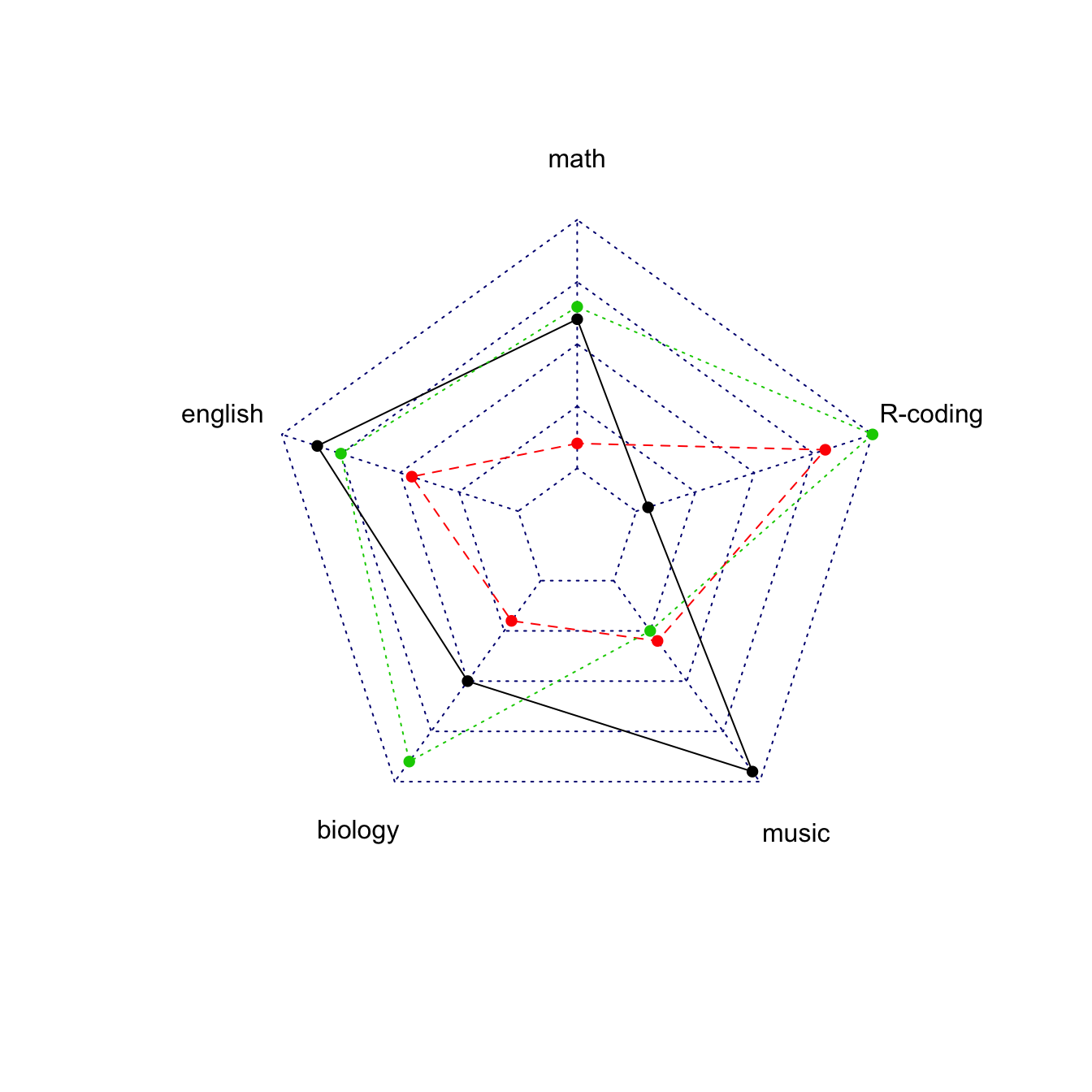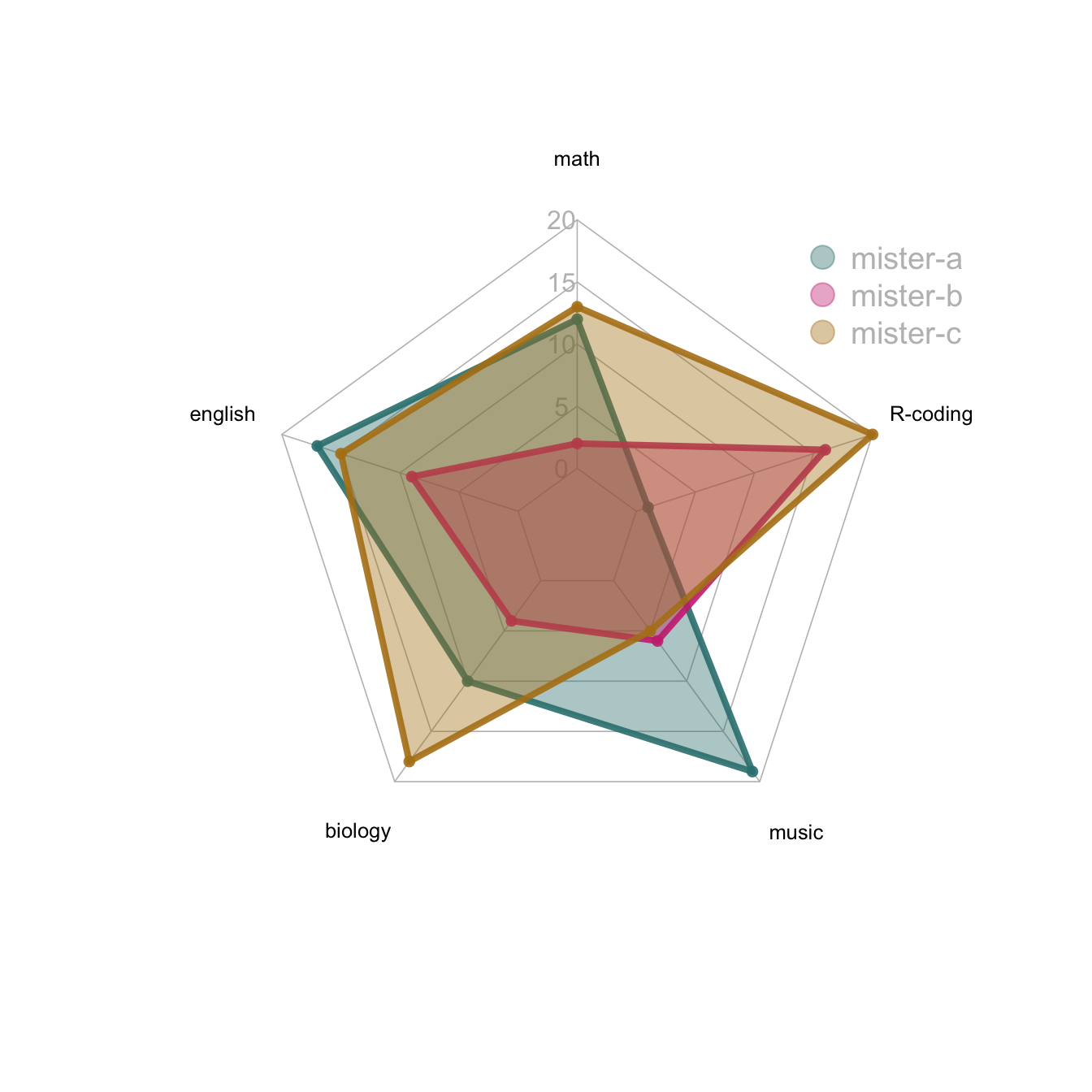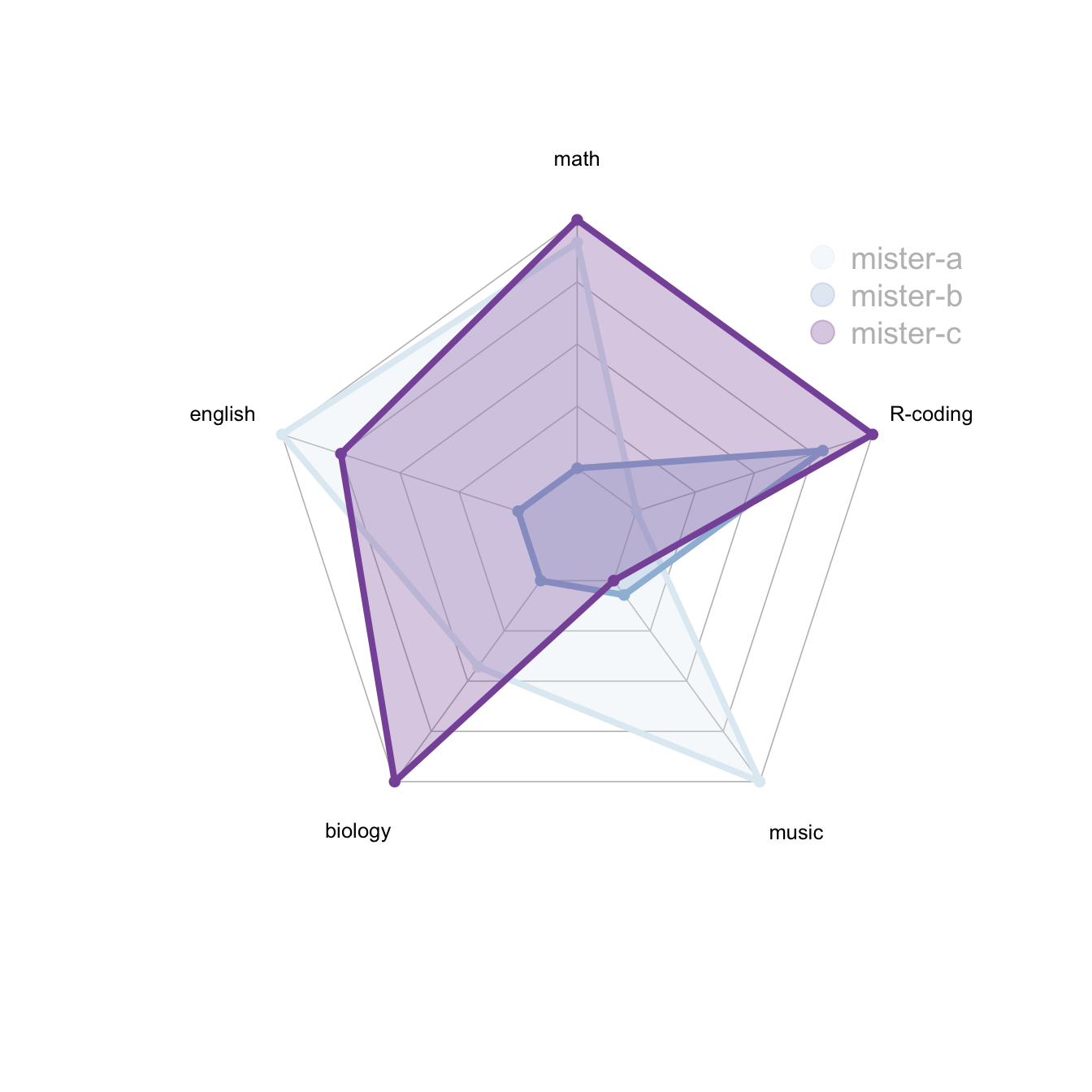# Radar chart with several individuals

It is possible to display several groups on the same radar chart. This post describes how to draw it with `R` and the `fmsb` library.

# Multi-group spider chart, no options

If you’re using the `fmsb` package for the first time, have a look to the most basic radar chart first, it explains how the data must be formatted for the `radarchart()` function.

If you have several individuals, the code looks pretty much the same as shown below.``````# Library
library(fmsb)

# Create data: note in High school for several students
set.seed(99)
data <- as.data.frame(matrix( sample( 0:20 , 15 , replace=F) , ncol=5))
colnames(data) <- c("math" , "english" , "biology" , "music" , "R-coding" )
rownames(data) <- paste("mister" , letters[1:3] , sep="-")

# To use the fmsb package, I have to add 2 lines to the dataframe: the max and min of each variable to show on the plot!
data <- rbind(rep(20,5) , rep(0,5) , data)

# plot with default options:

# Customization

The `radarchart()` function offers several options to customize the chart:

• Polygon features:
• `pcol` → line color
• `pfcol` → fill color
• `plwd` → line width

• Grid features:
• `cglcol` → color of the net
• `cglty` → net line type (see possibilities)
• `axislabcol` → color of axis labels
• `caxislabels` → vector of axis labels to display
• `cglwd` → net width

• Labels:
• `vlcex` → group labels size``````# Library
library(fmsb)

# Create data: note in High school for several students
set.seed(99)
data <- as.data.frame(matrix( sample( 0:20 , 15 , replace=F) , ncol=5))
colnames(data) <- c("math" , "english" , "biology" , "music" , "R-coding" )
rownames(data) <- paste("mister" , letters[1:3] , sep="-")

# To use the fmsb package, I have to add 2 lines to the dataframe: the max and min of each variable to show on the plot!
data <- rbind(rep(20,5) , rep(0,5) , data)

# Color vector
colors_border=c( rgb(0.2,0.5,0.5,0.9), rgb(0.8,0.2,0.5,0.9) , rgb(0.7,0.5,0.1,0.9) )
colors_in=c( rgb(0.2,0.5,0.5,0.4), rgb(0.8,0.2,0.5,0.4) , rgb(0.7,0.5,0.1,0.4) )

# plot with default options:
#custom polygon
pcol=colors_border , pfcol=colors_in , plwd=4 , plty=1,
#custom the grid
cglcol="grey", cglty=1, axislabcol="grey", caxislabels=seq(0,20,5), cglwd=0.8,
#custom labels
vlcex=0.8
)

legend(x=0.7, y=1, legend = rownames(data[-c(1,2),]), bty = "n", pch=20 , col=colors_in , text.col = "grey", cex=1.2, pt.cex=3)``````

In the previous examples, axis limits were set in the 2 first rows of the input dataset.

If you do not specify these values, the axis limits will be computed automatically, as shown below.``````# Library
library(fmsb)

# Create data: note in High school for several students
set.seed(99)
data <- as.data.frame(matrix( sample( 0:20 , 15 , replace=F) , ncol=5))
colnames(data) <- c("math" , "english" , "biology" , "music" , "R-coding" )
rownames(data) <- paste("mister" , letters[1:3] , sep="-")

# To use the fmsb package, I have to add 2 lines to the dataframe: the max and min of each variable to show on the plot!
data <- rbind(rep(20,5) , rep(0,5) , data)

# Set graphic colors
library(RColorBrewer)
coul <- brewer.pal(3, "BuPu")
colors_border <- coul
library(scales)
colors_in <- alpha(coul,0.3)

# If you remove the 2 first lines, the function compute the max and min of each variable with the available data:
radarchart( data[-c(1,2),]  , axistype=0 , maxmin=F,
#custom polygon
pcol=colors_border , pfcol=colors_in , plwd=4 , plty=1,
#custom the grid
cglcol="grey", cglty=1, axislabcol="black", cglwd=0.8,
#custom labels
vlcex=0.8
)

legend(x=0.7, y=1, legend = rownames(data[-c(1,2),]), bty = "n", pch=20 , col=colors_in , text.col = "grey", cex=1.2, pt.cex=3)``````

# Warning

There is a lot of criticism going around spider chart. Before using it in a project, you probably want to learn more about it.

Related chart types

## Contact

This document is a work by Yan Holtz. Any feedback is highly encouraged. You can fill an issue on Github, drop me a message on Twitter, or send an email pasting yan.holtz.data with gmail.com.